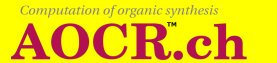# 2. The Computation Tool ( Web version )

### 2.2. Options

The MAIN list sets the computation mode. Choose start to begin a new reaction computation. The computation executes correctly only if a list of reaction step rules, a formula for the start compounds and a formula for the searched compounds have been saved with the corresponding selections of the Graphic Composer. Refer to 2.1 Basic facts.

Choose continue to proceed with the previous one at the previous position, by maintaining also the list of rules and the searched compounds.

The following computation parameters are all optional.

• TWO STEPS IN ONE: Check the box to make a computation step the result of two consecutive reaction step rule applications. The computation proceeds faster, but it skips the reaction step levels 1, 3, 5,... Therefore there is the possibility that the searched product is not found.
• GENERATION BOUND: If the GENERATION LEVELS are set, this option has no effect. Otherwise, it sets the highest index of a generated product from which the generation will occur. This option is a way (GENERATION LEVELS is another one) to limit the length of the calculation. Since continuation is possible there is no need to do a big chunk at one time. When GENERATION BOUND is set to a number, all formulas will be generated from a formula with a lower number than the one set. For example if GENERATION BOUND is set to 1, generation will happen only from the formula of the start compounds (that has number 0). If it is set to 10, formulas will be generated only from the first 10 (0-9) obtained products.